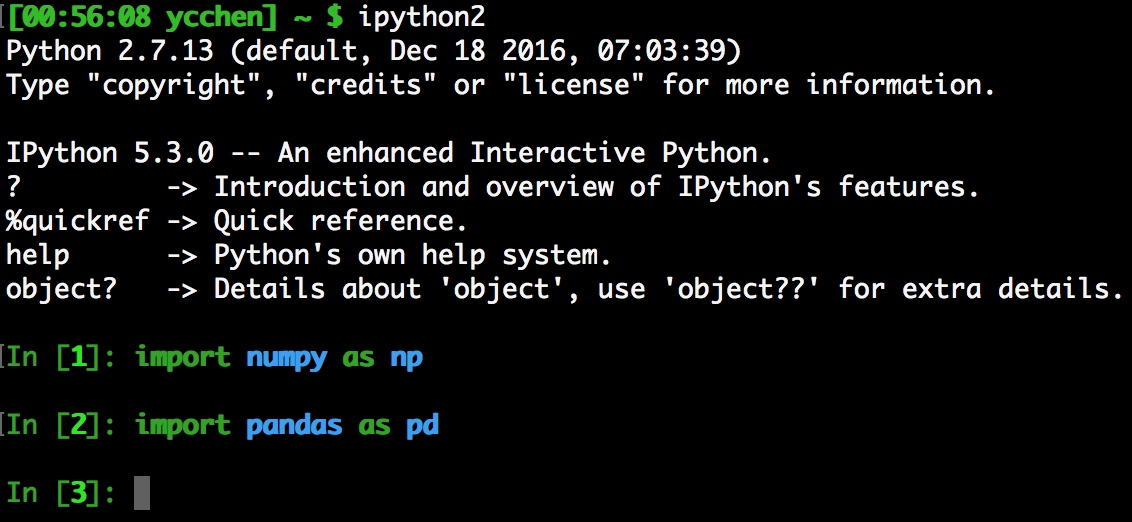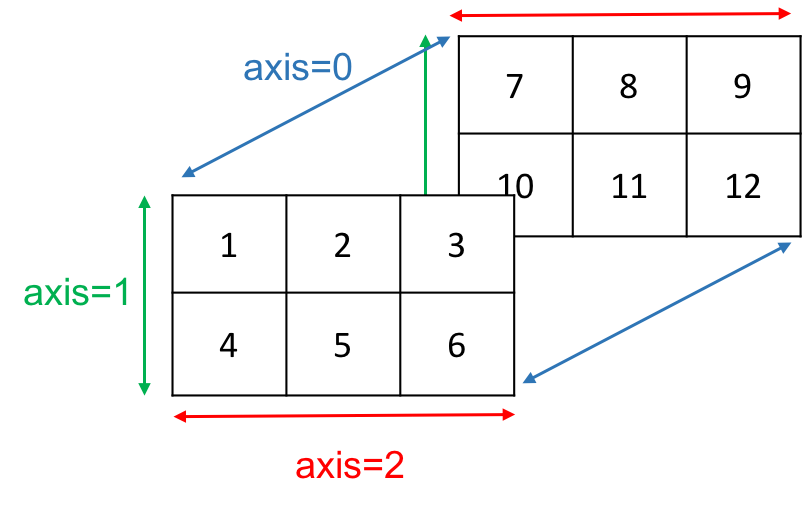# Python玩數據 (2)：Numpy [1/2]

Posted on April 17, 2017 in CS. View: 13,448

### IPythonIPython是一個具有互動式介面的python執行介面，你可以一邊寫一邊理解目前的狀況，舉個例子

 ```1 2 3``` ``````>>> a = 12 # integer(整數) >>> a # check variable a 12 ``````

### Python常見的資料型別

Python常見的資料型別有整數(integer)、浮點數(floating-point number)、字串(string)、串列(list)、序對(tuple)、字典(dictionary)，可以使用`type()`來查詢資料型別。

 ``` 1 2 3 4 5 6 7 8 9 10 11 12``` ``````>>> a = 10 # integer >>> b = 40.0 # float, 必須有'.' >>> c = 'word' # string >>> d = [1, 2.0, '3'] # list >>> e = (4, 5.0, '6') # tuple >>> f = {'a':1, 'b':2 } # dictionary >>> type(c) str >>> type(d) list >>> type(f) dict ``````

list和tuple裡面可以塞入任意的資料型別，甚至可以塞入另外一個list，或是自己定義的物件，list和tuple其實非常的相似，差異只在於tuple一旦決定了就不能在變更，但是list卻可以。

 ```1 2 3 4 5 6 7 8 9``` ``````>>> d.append('new') >>> d [1, 2.0, '3', 'new'] >>> d = d + 1 # 取出第一項(index=0)加一再設定回去第一項 >>> d [2, 2.0, '3', 'new'] >>> del d # 刪除index=1的那項 >>> d [2, '3', 'new'] ``````
 ```1 2 3 4 5 6 7 8``` ``````>>> e.append('new') # fail AttributeError: 'tuple' object has no attribute 'append' >>> e 4 >>> e = e + 1 # fail TypeError: 'tuple' object does not support item assignment >>> del e # fail TypeError: 'tuple' object doesn't support item deletion ``````

 ```1 2 3 4 5 6``` ``````>>> 1 + 2 * 2 - 6 / 2 2 >>> 1.0 + 2.0 * 2.0 - 6.0 / 2.0 2.0 >>> 3 ** 2 # 指數 9 ``````

 ```1 2 3 4 5 6``` ``````>>> 1 / 2 0 # 整數除整數，結果必定是整是，是整數0，而不是想像中的0.5，這種運算效果有點像求商 >>> 1 / 2. 0.5 # 只要有任意浮點數出現，整數強迫轉為浮點數，然後再做運算，這才是我們要的結果 >>> 3 ** 2. 9.0 ``````

 ```1 2 3 4``` ``````>>> 1 + '2' # fail TypeError: unsupported operand type(s) for +: 'int' and 'str' >>> 1 + int('2') # 使用int()將字串轉成整數 3 ``````

 ```1 2 3 4 5 6 7``` ``````>>> f = (1,2,3) >>> h = list(f) >>> h [1, 2, 3] >>> h.insert(0,4) # 在index為0的地方插入整數4 >>> h [4, 1, 2, 3] ``````

### Numpy的數學運算

 ``` 1 2 3 4 5 6 7 8 9 10 11 12``` ``````>>> np.sum([1,2,3]) # 加總 6 >>> np.max([1,2,3]) # 最大值 3 >>> np.min([1,2,3]) # 最小值 1 >>> np.mod(5,2) # 求餘數 1 >>> np.sin(np.pi/2.) # 求sin 1.0 >>> np.log(np.exp(1)) # ln 和 e 1.0 ``````

### Numpy基礎元素：ndarray

Numpy最重要的元素就是ndarray，它是N-Dimensional Array的縮寫，在Numpy裡，dimesions被稱為axes，而axes的數量被稱為rank，axes是一個重要的概念，了解這個概念基本上就把Numpy搞懂一半以上了。

 ```1 2 3``` ``````>>> A = np.array([1,2,3]) >>> A array([1, 2, 3]) ``````

 ```1 2 3 4 5 6``` ``````>>> np.sum(A,axis=None) # axis為None的時候則加總所有元素 6 >>> np.sum(A,axis=0) 6 >>> np.sum(A,axis=1) # fail 因為A只有一維 ValueError: 'axis' entry is out of bounds ``````

 ```1 2``` ``````>>> np.sum(A,axis=-1) 6 ``````

 ```1 2 3 4 5 6 7 8``` ``````>>> B = np.array([[1,2,3],[4,5,6]]) >>> B array([[1, 2, 3], [4, 5, 6]]) >>> np.sum(B,axis=0) array([5, 7, 9]) # [1+4, 2+5, 3+6] >>> np.sum(B,axis=1) array([ 6, 15]) # [1+2+3, 4+5+6] ``````

 ```1 2 3 4 5 6``` ``````>>> np.sum(B) 21 >>> np.sum(B, axis=None) # same as above 21 >>> np.sum(B, axis=-1) # 和axis=1等價 array([ 6, 15]) ``````

 ``` 1 2 3 4 5 6 7 8 9 10 11 12 13 14 15 16``` ``````>>> C = np.array([[[1,2,3],[4,5,6]],[[7,8,9],[10,11,12]]]) >>> C array([[[ 1, 2, 3], [ 4, 5, 6]], [[ 7, 8, 9], [10, 11, 12]]]) >>> np.sum(C,axis=0) array([[ 8, 10, 12], # [1+7, 2+8, 3+9 ] [14, 16, 18]]) # [4+10, 5+11, 6+12] >>> np.sum(C,axis=1) array([[ 5, 7, 9], # [1+4, 2+5, 3+6] [17, 19, 21]]) # [7+10, 8+11, 9+12] >>> np.sum(C,axis=2) array([[ 6, 15], # [1+2+3, 4+5+6] [24, 33]]) # [7+8+9, 10+11+12] `````````1 2 3 4 5 6 7``` ``````>>> D = np.array([[1,2],[3,4],[5,6]]) >>> D array([[1, 2], [3, 4], [5, 6]]) >>> D.shape (3, 2) ``````

`(3, 2)`這樣的shape我們就一點都不意外了，axis=0有三個元素，而axis=1有兩個元素。shape可以直接改，如果數量恰當的話就會自動重組。

 ```1 2 3 4 5``` ``````>>> D.shape = (2,1,3) >>> D array([[[1, 2, 3]], [[4, 5, 6]]]) ``````

axis=0有兩個元素，axis=1有一個元素，axis=2有三個元素。

 ```1 2 3 4``` ``````>>> D[1, 0, 1] 5 >>> D[0, 0, 2] 3 ``````

ndarray有其資料型別，這個資料型別就稱為dtype，有哪些內建的資料型別呢？我們可以透過numpy的內建資料來查看。

 ```1 2 3 4 5 6``` ``````>>> np.sctypes {'complex': [numpy.complex64, numpy.complex128, numpy.complex256], 'float': [numpy.float16, numpy.float32, numpy.float64, numpy.float128], 'int': [numpy.int8, numpy.int16, numpy.int32, numpy.int64], 'others': [bool, object, str, unicode, numpy.void], 'uint': [numpy.uint8, numpy.uint16, numpy.uint32, numpy.uint64]} ``````

 ``` 1 2 3 4 5 6 7 8 9 10``` ``````>>> t1 = np.array([1,2,3],dtype='int32') >>> t1 array([1, 2, 3], dtype=int32) >>> t1.dtype dtype('int32') >>> t2 = np.array([1,2,3],dtype='float64') >>> t2 array([ 1., 2., 3.]) >>> t2.dtype dtype('float64') ``````

### Numpy的矩陣運算

 ```1 2 3 4 5``` ``````>>> A = np.array([[1,2],[3,4]],dtype='float64') >>> B = np.array([[5,0],[0,0]],dtype='float64') >>> A+B # element-wise plus array([[ 6., 2.], [ 3., 4.]]) ``````

A和B矩陣中同樣位置的元素相加，再放到新的矩陣中，這一種操作就叫做element-wise operation。

 ```1 2 3 4 5 6 7 8 9``` ``````>>> A-B # element-wise minus array([[-4., 2.], [ 3., 4.]]) >>> A*B # element-wise multiply array([[ 5., 0.], [ 0., 0.]]) >>> B/A # element-wise divide array([[ 5., 0.], [ 0., 0.]]) ``````

 ```1 2 3``` ``````>>> np.dot(A,B) # 矩陣內積 array([[ 5., 0.], [ 15., 0.]]) ``````

 ```1 2 3 4 5 6 7``` ``````>>> A = np.array([[1,2],[3,4]],dtype='float64') >>> A array([[ 1., 2.], [ 3., 4.]]) >>> A.T # 矩陣轉置 array([[ 1., 3.], [ 2., 4.]]) ``````

 ```1 2 3 4 5 6 7``` ``````>>> A_rev = np.linalg.inv(A) # 反矩陣 >>> A_rev array([[-2. , 1. ], [ 1.5, -0.5]]) >>> np.dot(A,A_rev) array([[ 1.00000000e+00, 0.00000000e+00], [ 8.88178420e-16, 1.00000000e+00]]) ``````

A和A的反矩陣內積為單位矩陣，你有注意到`8.88178420e-16`這個奇怪的數字嗎？這是因為python在計算的過程有一些誤差的緣故，所以才會產生一個這麼小的數字，但基本上可以看作是0。

 ```1 2 3 4 5 6 7 8``` ``````>>> A = np.array([[1,2],[3,4]],dtype='float64') >>> B = np.array([[5,0],[0,0]],dtype='float64') >>> V = np.vstack((A,B)) >>> V array([[ 1., 2.], [ 3., 4.], [ 5., 0.], [ 0., 0.]]) ``````

 ```1 2 3 4``` ``````>>> H = np.hstack((A,B)) >>> H array([[ 1., 2., 5., 0.], [ 3., 4., 0., 0.]]) ``````

 ```1 2 3 4``` ``````>>> np.vsplit(V,2) # 2代表切兩份 [array([[ 1., 2.], [ 3., 4.]]), array([[ 5., 0.], [ 0., 0.]])] ``````

 ```1 2 3 4 5 6``` ``````>>> np.hsplit(H,4) # 4代表切四份 [array([[ 1.], [ 3.]]), array([[ 2.], [ 4.]]), array([[ 5.], [ 0.]]), array([[ 0.], [ 0.]])] ``````

### 子彈總結

• Python常見的資料型別：整數(integer)、浮點數(floating-point number)、字串(string)、串列(list)、序對(tuple)、字典(dictionary)
• ndarray的axes概念很重要，這會決定函數操作的方式，例如：np.sum
• ndarray的資料型別(dtype)，例如：'float64', 'int64', 'string', ...
• numpy的矩陣運算有element-wise operation和matrix operation兩種